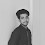# URI - BEECROWD - BEE Online Judge Solution  1006 | Average 2 - URI - BEECROWD - BEE Problem 1006 Solution in C,C++,Python

Read three values (variables A, B and C), which are the three student's grades. Then, calculate the average, considering that grade A has weight 2, grade B has weight 3 and the grade C has weight 5. Consider that each grade can go from 0 to 10.0, always with one decimal place.

## Input

The input file contains 3 values of floating points with one digit after the decimal point.

## Output

Print the message "MEDIA"(average in Portuguese) and the student's average according to the following example, with a blank space before and after the equal signal.

 Input Samples Output Samples 5.06.07.0 MEDIA = 6.3
 5.010.010.0 MEDIA = 9.0
 10.010.05.0 MEDIA = 7.5

### URI Online Judge Solution  1006 | Average 2 - URI Problem 1006 Solution in C,C++,Python::

URI Problem 1006 Solution in C :

### 2 Responses to URI - BEECROWD - BEE 1006 | Average 2 - Solution in C,C++,Python

1.is there any one who can teach the solution why we need to devide a b c by 10

2.Because the total weight of a+b+c is 2+3+5 = 10